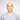# Previous Page Token for Cursor Pagination

#### a.k.a. KeySet or Seek PaginationPostgreSQL B-Tree Index Explained

Before we begin, it’s important to note that the need for previous page traversal can be eliminated through GUI (graphical user interface) designs that don’t even include a Previous Page button. Infinity Scroll is one example. Another is machine-interfacing designs where the client machine just wants to run through the entire result set. If Cursor Pagination is an unfamiliar topic, please visit the following posts, as this one is intended as an extension:

The first link does a good job explaining how to implement previous page traversal, however, a different look could help some people understand what’s happening a little better.

## The Problem

Cursor Pagination SQL queries rely on indexed columns in conjunction with comparison operators, rather than an `OFFSET` clause. This is straight-forward when traversing the next pages of an incrementing (`ASC`) result set:

``SELECT * FROM entries WHERE (myCol > 5) ORDER BY myCol ASC LIMIT 2;``Next page:

``SELECT * FROM entries WHERE (myCol > 7) ORDER BY myCol ASC LIMIT 2;``This is also straight-forward when traversing the next pages of a decrementing (`DESC`) result set:

``SELECT * FROM entries WHERE (myCol < 5) ORDER BY myCol DESC LIMIT 2;``Next page:

``SELECT * FROM entries WHERE (myCol < 3) ORDER BY myCol DESC LIMIT 2;``However, traversing previous pages becomes tricky, because when you provide a constraint in an SQL query (e.g. `>`, `<`), SQL Database engines (e.g. Postgres) return the left-most results:

``SELECT * FROM entries WHERE (myCol < 8) ORDER BY myCol ASC LIMIT 2;``We wanted 6 and 7!

## The Solution

The solution for properly implementing previous page traversal is to reverse the ordering of your result set (ASC vs DESC) so that the results you want are to the right of the cursor. And then once the LIMIT is applied, reverse the ordering again so that it matches the ordering that requested:

``SELECT pagination.* FROM(SELECT * FROM entries WHERE (myCol < 8) ORDER BY myCol DESC LIMIT 2) AS pagination ORDER BY myCol ASC;``

Note: A CTE (common table expression) would also work as an alternative to nested queries.Previous page again:

``SELECT pagination.* FROM(SELECT * FROM entries WHERE (myCol < 6) ORDER BY myCol ASC LIMIT 2) AS pagination ORDER BY myCol DESC;``## Time Complexity Analysis

The performance overhead of previous page traversal compared to next page traversal is negligible — it’s the difference between `O(log(N) + 2L)` vs `O(log(N) + L)` where `N` is the size of the table and `L` is the size of the limit (technically, `L` could be equal to `N` if `N <= LIMIT`). Furthermore, `L` could even be considered a constant, rather than an unbounded variable since page size can only be so large before it quickly breaches message/payload size limits. `Log(N)` is the time it takes the DB engine to traverse the index tree from the root node to the leaf node that satisfies the `WHERE` clause. In scenarios where `L` is very small (less than 5) and `N` is very large (millions), `log(N)` could be greater than `L`. It’s important to note that no sorting is required in these queries since the index already maintains the data in a sorted manner (in the leaf nodes of the index tree). In previous page traversal, a second scan equal to the size of the limit is required to achieve the desired order, due to the quirk mentioned above.

### Authors